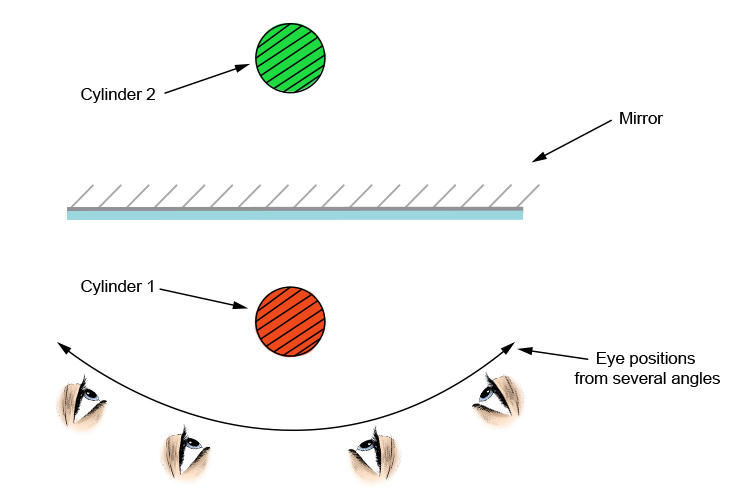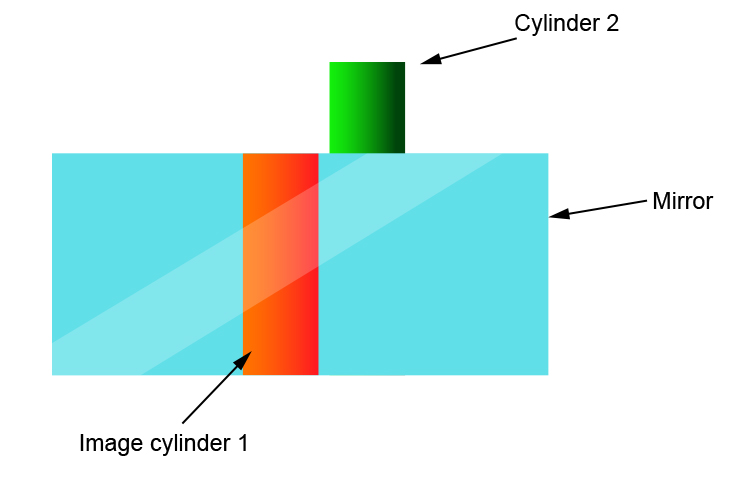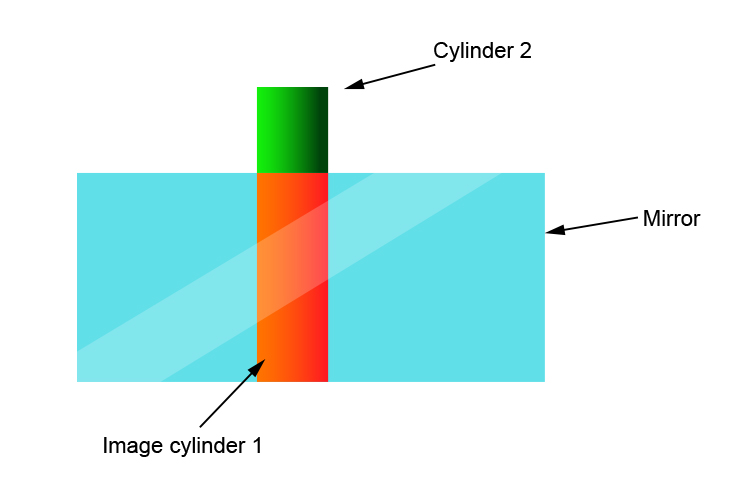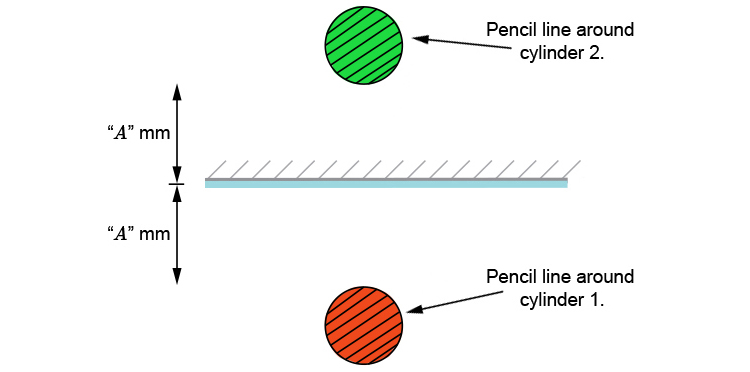# Plane mirrors and parallax experiment

We know from our ray diagram that the image is

Behind the mirror
Equal distance
Same size

but we can find the location of the image using parallax.

## The experiment

Mark the position of a mirror that is standing in a vertical position.

Have two identical objects that are taller than the mirror. In this case we will use two thin cylinders.

Put the two cylinders either side of the mirror and look at the image created from several angles:What you will probably see in the mirror is the following. Cylinder 2 and cylinder 1 will move around as you move your head from side to side.You have to adjust cylinder 2 until the two cylinders stay together.

What you need to get to is as below:The two images should be in perfect parallel position not a parallel lax position (parallax) or in other words they should not be exhibiting any parallax.

When there is no parallax you can then mark around each of the cylinders with a pencil and you should get the following:You can then measure the distance between the cylinders from the mirror and they will be the same.

So we can now prove that the image in a plane mirror is:

Behind the mirror
Equal distance
and Same size